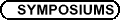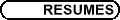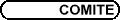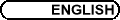Special Structures in Differential Geometry / Structures spéciales en géométrie différentielleOrg: Gordon Craig (Bishop's) and/et Spiro Karigiannis (McMaster) VESTISLAV APOSTOLOV, UQAM, 201 av. Président Kennedy, Montréal (QC), Canada Extremal Kähler metrics and Hamiltonian 2-forms I will introduce the notian of a Hamiltonian 2-form on a Kähler manifold, and present classification results for compact Kähler manifolds admitting such forms. These can be used as a tool of obtaining new explicit examples of extremal Kähler metrics on certain projective bundles. This is a joint work with D. Calderbank, P. Gauduchon and C. Tønnesen-Friedman. HENRIQUE BURSZTYN, University of Toronto and Fields Institute, 100 St. George Street, Toronto, ON M5S 3G3 Dirac structures and symmetries in symplectic geometry Dirac structures (introduced by T. Courant and A. Weinstein about 15 years ago) generalize, simultaneously, closed 2-forms, Poisson structures, and regular foliations. The talk will discuss the geometry of Dirac manifolds and show how Dirac geometry provides a natural setting for the study of generalized notions of symmetries in symplectic geometry (including the theory of quasi-hamiltonian spaces and Lie group-valued moment maps due to Alekseev, Malkin and Meinrenken). ADRIAN BUTSCHER, University of Toronto Construction of Special Legendrian Surfaces of the Sphere of Higher Genus A method for constructing special Lagrangians cones in C3 whose links are higher-genus special Legendrian surfaces in S5 is to form minimal and Legendrian connected sums of multiple copies of \operatornameSU(3)-rotated Clifford tori in S5, and then to consider the cones over these objects. There are two algebraic conditions on a collection of proposed \operatornameSU(3)-rotations, such that when these conditions hold, the construction can be carried out using a geometric PDE argument known as gluing. Furthermore, a number of examples of collections of \operatornameSU(3)-rotations will be studied for which the conditions do not hold, and the possibility of finding a collection of \operatornameSU(3)-rotations for which the conditions do hold will be discussed. BENOIT CHARBONNEAU, McGill University, Montreal Analytic aspects of periodic instantons Instantons are particular connections on four-manifolds, satisfying a partial differential equation called the anti-self-dual equation. In principle, there exist a correspondence between instantons on a quotient of R4 by a translation symmetry subgroup and the solutions to a related equation on a quotient by some dual subgroup. This correspondence, a non-linear analog of the Fourier transform, is called the Nahm transform and provides a better understanding of the spaces of instantons. GORDON CRAIG, Bishop's University, Lennoxville, Quebec Dehn Fillings and Asymptotically Hyperbolic Einstein Manifolds I will describe a gluing construction which allows one to fill in the cusps of certain infinite-volume, noncompact hyperbolic manifolds in such a way as to obtain infinitely many asymptotically hyperbolic Einstein manifolds with a shared conformal infinity. DAVID DUCHEMIN, UQAM, C.P. 8888, Centre-ville, Montréal (Québec), H3C 1P8 Quaternionic contact structures in dimension 7 The conformal infinity of a quaternionic-Kähler metric on a 4n-manifold with boundary is a codimension 3-distribution on the boundary called quaternionic contact. I will describe this contact geometry and its links with special riemannian geometry. Especially, I prove a criterion for quaternionic contact structures on 7-dimensional manifolds to be the conformal infinity of a quaternionic-Kähler metric, although there is no obstruction in greater dimensions. MARCO GUALTIERI, Fields Institute, Toronto Generalized geometry I will provide an introduction to the new field of generalized geometry, initiated by Hitchin (math.DG/0209099) and developed in my thesis (math.DG/0401221). I will concentrate mainly on generalized complex geometry, which is a unification of complex and symplectic geometry. I will make some comments about the implications for mirror symmetry. If time permits I will speak about generalized Riemannian and Kahler geometry. PENGFEI GUAN, McGill University Conformal deformation of Ricci tensor We report a recent work in conformal geometry jointly with G. Wang. The lower bound of Ricci curvature tensor plays an important role in Riemannian geometry. The problem we are interested is the conformal deformation of the smallest eigenvalue of the Ricci tensor. The problem is equivalent to solving an interesting fully nonlinear equation which is uniformly elliptic, but the operator is only Lipschitz. The equation is similar to the Yamabe equation and we encounter a similar non-compactness difficulty. We use some geometry, e.g., Aubin and Schoen's resolution of the Yamabe problem and the Bishop-Gromov volume comparison theorem. And in analysis part, we establish the local gradient estimates and make use of Caffarelli's fundamental C2,a estimates for these type of fully nonlinear equations. We will also discuss geometrical applications. MARIANTY IONEL, McMaster University Families of Calibrated 4-folds I will discuss a result of mine in classifying the special Lagrangian 4-folds in C4 whose second fundamental form has a non-trivial SO(4)-stabilizer at each point. Points on special Lagrangian 4-folds where this stabilizer is nontrivial are the analogs of the umbilical points in the classical theory of surfaces. I will also talk about the case of Cayley 4-folds in R8 with a certain pointwise symmetry on their second fundamental form, which is work in progress. NIKY KAMRAN, McGill University, Montreal, Canada Decay of scalar waves in Kerr geometry I shall report on some significant recent progress in the study of the long term behavior of scalar waves in the Kerr geometry of a rotating black hole in equilibrium, including decay theorems. This is joint work with Felix Finster, Joel Smoller and Shing-Tung Yau. SPIRO KARIGIANNIS, McMaster University Properties of Moduli Spaces of G2 Metrics I will discuss some properties of the moduli space of G2-metrics on a G2-manifold, emphasising the relations and differences with the properties of the moduli space of Calabi-Yau metrics. This is a preliminary report on joint work with Nai-Chung Conan Leung. MAUNG MIN-OO, McMaster University, Hamilton, ON L8S 4K1 Calibrated cycles in vector bundles with special holonomy In this talk we will report on a simple new way of constructing calibrated cycles in certain vector bundles over the sphere and complex projective space equipped with metrics of special holonomy discovered by Calabi, Stenzel, Bryant and Salamon. This is joint work with S. Karigiannis. We will also explain, in the G2 and Spin(7) case, how the metrics and the cycles are related to each other as circle lifts. RUXANDRA MORARU, Fields Institute Hypercomplex structures on instanton moduli spaces In this talk, we describe how hypercomplex structures arise on moduli spaces of instantons over locally conformally hyperkähler manifolds. We also discuss how these hypercomplex structures relate to the natural Poisson structures that exist on the moduli. This is work in progress.